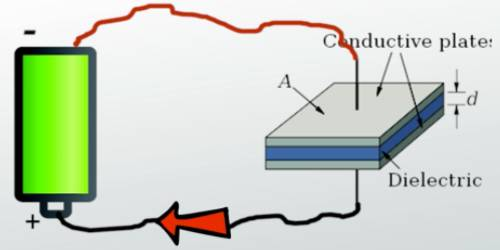# Capacitance of Capacitor

Capacitance of Capacitor

Capacitance is the ability to store electrical energy. Almost all things, including you, can store some electrical energy and therefore have capacitance. When you rub your feet across a carpet, charged particles called electrons can be transferred from the carpet to you.

Generally, a capacitor consists of the combination of an insulated conductor and a grounded conductor. So, the capacitance of capacitor means the capacitance of the insulated conductor and it can be defined as –

“The amount of charge given to an insulated conductor in order to create a unit potential difference between the insulated conductor and a grounded conductor is called capacitance of the capacitor.”

The capacitance of a capacitor

= [Charge of an insulated conductor / Potential difference between the two conductors]

The capacitance of a capacitor depends on the size and shape of the two conductors and the distance and the medium between the conductors.

“Capacitance of a capacitor is 2F” – means that in order to produce 1 volt of potential difference between the two plates 2 coulomb of charge is to be given to the insulated conductor.

Capacitance is defined as being that a capacitor has the capacitance of One Farad when a charge of One Coulomb is stored on the plates by a voltage of One volt. Note that capacitance, C is always positive in value and has no negative units.